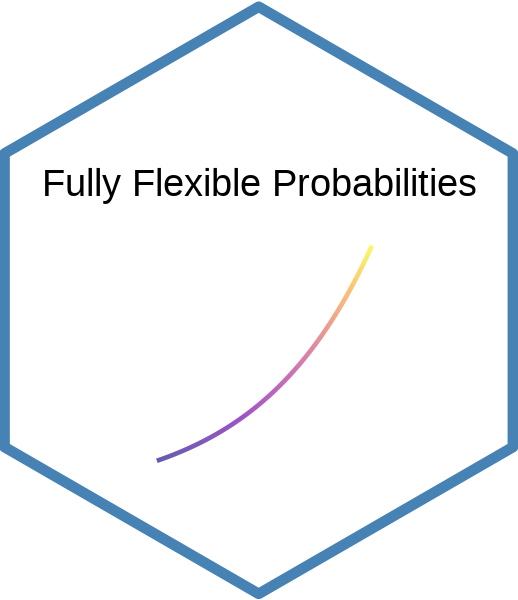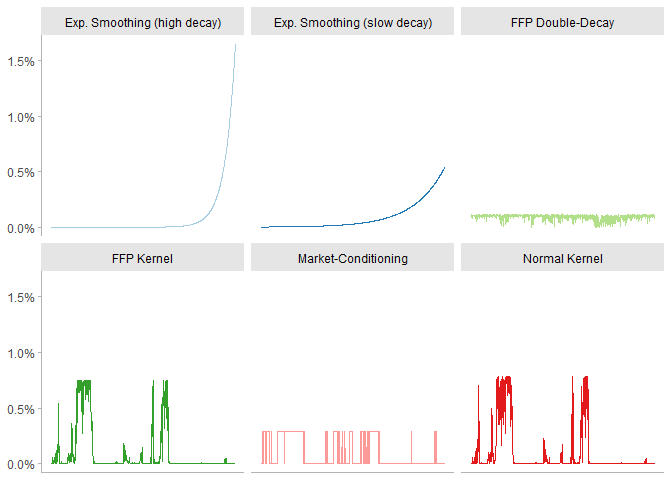# Fully Flexible Probabilities

Functions for Scenario Analysis and Risk Management

Oftentimes, the econometrician needs to stress-test the potential outcomes for a given set of risk-drivers. This process can be computationally costly when the entire set of scenarios needs to be repriced.

To overcome this difficulty, the Fully Flexible Probabilities (FFP) approach offers an inexpensive way for scenario generation: it reprices the probabilities associated to each scenario, instead of the scenarios themselves. Once the new probabilities have been defined, the computations can be performed very quickly because the burden of scenario generation has been left aside.

## Installation

Install the official version from CRAN with:

``install.packages("ffp")``

Install the development version from github with:

``````# install.packages("devtools")
devtools::install_github("Reckziegel/ffp")``````

## Probability Estimation

The package `ffp` comes with five functions to extract probabilities from the historical scenarios:

• `exp_decay()`: accounts for the time-changing nature of volatility by giving more weight to recent observations;
• `crisp()`: selects scenarios where a logical statement is satisfied;
• `kernel_normal()`: generalizes the `crisp` condition by wrapping scenarios over a normal kernel;
• `kernel_entropy()`: uses entropy-polling to satisfy a conditioning statement;
• `double_decay()`: uses entropy-polling and a double-decay factor to constrain the first two moments of a distribution.## Stress-Testing and Portfolio Construction

The package also offers eight different constructors to make it easier to input views on the market for portfolio optimization (mean-variance, risk-parity, etc.):

• `view_on_mean()`
• `view_on_covariance()`
• `view_on_correlation()`
• `view_on_volatility()`
• `view_on_rank()`
• `view_on_copula()`
• `view_on_marginal_distribution()`
• `view_on_joint_distribution()`

The output is a list that `entropy_pooling()` can handle easily. To combine multiple views in a single object use `bind_views()`.

## Scenario Analysis

Once the new probabilities have been estimated, `bootstrap_scenarios()` can be used to sample data, while keeping the structure of the empirical copulas intact.

The main statistics of arbitrary scenarios can be computed with `empirical_stats()`.